Web www.handwritingforkids.com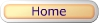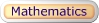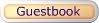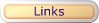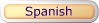### Online Math 1 - Subtraction Math Drill/Test (Vertical)

This online/interactive generator will provides plenty of math drill and practice in subtraction, to boost test scores, improves accuracy and speed to prepare the child/children to meet the Kindergarten, Grade 1, and Grade 2 curriculum standards. This online/interactive math subtraction, will create 1 digit by 1 digit (1x1) and 2 digits by 1 digit (2x1) equations with an option of timed test, and it will automatically scored your results in a printable worksheet.

Type of online math problems:

• Online Math 1 - Subtraction - Difference 0 to 5
• Online Math 1 - Subtraction - Difference 5 to 10
• Online Math 1 - Subtraction - Difference 0 to 10
• Online Math 1 - Subtraction - Difference 0 to 18
• Online Math 1 - Subtraction - Difference 10 to 18

 6 - 5 1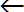minuendsubtrahenddifference

2. Select the number of math problems per page. (Default: 1 - 20)
3. Select Type of Math Problems. (Default: Difference 0 to 10)
4. Do you want a timed-test? (Default: Yes)
5. Click on submit to make your personalized online vertical math subtraction drill/test.
6. Option: Page back and click Submit to create a different set of math problems.

Online Math 1 - Subtraction Math Drill/Test Maker (Vertical)

 Name: (optional)

Enter the Number of Math Problems per page:

Default: 1 - 20

Type of Math Problems:

Default: Difference 0 to 10

Yes, Timed-Test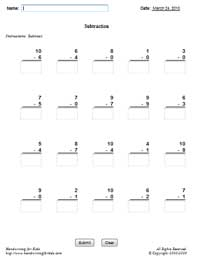Basic Handwriting for Kids Free for Non-Profit Use Ms. Readman, Rockford, IL Revised: